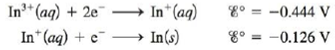# The following standard reduction potentials have been determined for the aqueous chemistry of indium: a. What is the equilibrium constant for the disproportionation reaction, where a species is both oxidized and reduced, shown below? 3 In + ( a q ) → 2 In ( s ) + In 3 + ( a q ) b. What is Δ G f ∘ for In + (aq) if Δ G f ∘ = −97.9 kJ/mol for In 3+ ( aq )?### Chemistry: An Atoms First Approach

2nd Edition
Steven S. Zumdahl + 1 other
Publisher: Cengage Learning
ISBN: 9781305079243

#### Solutions

Chapter
Section### Chemistry: An Atoms First Approach

2nd Edition
Steven S. Zumdahl + 1 other
Publisher: Cengage Learning
ISBN: 9781305079243
Chapter 17, Problem 155IP
Textbook Problem
28 views

## The following standard reduction potentials have been determined for the aqueous chemistry of indium:a. What is the equilibrium constant for the disproportionation reaction, where a species is both oxidized and reduced, shown below? 3 In + ( a q ) → 2 In ( s ) + In 3 + ( a q ) b. What is Δ G f ∘ for In+(aq) if Δ G f ∘ = −97.9 kJ/mol for In3+(aq)?

(a)

Interpretation Introduction

Interpretation: The equilibrium constant for the given disproportionation reaction and the standard Gibbs free energy of formation ΔGfο are to be calculated.

Concept introduction: Equilibrium constant is defined as the ratio of the concentration of products and the concentration of the reactants and Gibbs free energy is a thermodynamic quantity that is used to calculate the maximum work of reversible reaction performed by a system. It is equal to the difference between the enthalpy and the product of entropy at absolute temperature.

To determine: The equilibrium constant for the given disproportionation reaction.

### Explanation of Solution

Explanation

The equilibrium constant for the given disproportionation reaction is K=5.77×1010_ .

Given

The half cell reactions for the cell are,

In3+(aq)+2eIn+(aq)                      E1ο=0.444 V (1)

In+(aq)+eIn(s)                           E2ο=0.126 V (2)

Where,

• E1ο is the standard electrode potential of equation (1).
• E2ο is the standard electrode potential of equation (2).

The balanced cell reaction obtained by reversing reaction (1) and then multiplying reaction (1) by 3 and reaction (2) by 2 is,

3In3+(aq)+3e3In+(aq)                      E2ο=0.126 V (3)

2In+(aq)2In(s)+2e                         E1ο=0.444 V (4)

The overall cell reaction is,

3In+(aq)+e2In(s)+In3+(aq) (5)

The standard electrode potential of equation (5) is calculated by the formula,

Ecellο=E2οE1ο

Where,

• Ecellο is the standard electrode potential of equation (3).

Substitute the values of E1ο and E2ο in the above formula

(b)

Interpretation Introduction

Interpretation: The equilibrium constant for the given disproportionation reaction and the standard Gibbs free energy of formation ΔGfο are to be calculated.

Concept introduction: Equilibrium constant is defined as the ratio of the concentration of products and the concentration of the reactants and Gibbs free energy is a thermodynamic quantity that is used to calculate the maximum work of reversible reaction performed by a system. It is equal to the difference between the enthalpy and the product of entropy at absolute temperature.

To determine: The standard Gibbs free energy of formation ΔGfο for the given disproportionation reaction.

### Still sussing out bartleby?

Check out a sample textbook solution.

See a sample solution

#### The Solution to Your Study Problems

Bartleby provides explanations to thousands of textbook problems written by our experts, many with advanced degrees!

Get Started

Find more solutions based on key concepts
A ________ is dissolved in a solvent. a. molecule b. salt c. solute d. chemical bond

Biology: The Unity and Diversity of Life (MindTap Course List)

B vitamins often act as: a. antioxidants. b. blood clotting factors. c. coenzymes. d. none of the above.

Nutrition: Concepts and Controversies - Standalone book (MindTap Course List)

A speedboat moving at 30.0 m/s approaches a no-wake buoy marker 100 m ahead. The pilot slows the boat with a co...

Physics for Scientists and Engineers, Technology Update (No access codes included)

What causes global warming?

Oceanography: An Invitation To Marine Science, Loose-leaf Versin